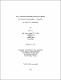## Development of interval and non-interval methods for solving multi-objective optimization problems2018
##### Publisher
University of Alabama Libraries
##### Abstract

Multi-objective optimization problems are pervasive across many diverse disciplines in engineering, economics, management and design where optimal decisions need to be made in the presence of tradeoffs between two or more conflicting objectives with respect to a set of decisions and subject to a set of constraints (Awad and Khanna 2015). The interval method (as a non-scalarization MOP method) is known as a precise and robust tool for optimization that guarantees to capture all points on the Pareto-front (Kubica and Woźniak 2007) . This research investigated the interval and non-interval methods for multi-objective optimization problems with or without constraints. The underlying framework for our improved interval methods are standard Hansen method and Big Cube and Small Cube method. Listed below are some strategies we have developed: a) Twin interval arithmetic is proposed in an effort to improve the quality of estimates for the range of functions. The main use of twin interval arithmetic (TIA) in the improved interval algorithms is to provide an extra choice for the cut-off value in the discarding process. b) Exclusion zone functions are introduced to further reduce variables' intervals which can be added as a reduction step in the standard Hansen method. c) For constrained global optimization problems with two linear constraints, we outlined a steepest descent procedure to locate a feasible sample point within the framework of Hansen method. d) A hybrid BCSC algorithm supported by the three above-mentioned strategies is described in Chapter 3. A refined sampling step is introduced to the BCSC algorithm and this algorithm was marked as original BCSC for comparison in Chapter 3. Numerical results with respect to each strategy are illustrated to show its effectiveness in Chapter 3. Three non-interval multi-objective linear programming (MOLPs) algorithms are presented in Chapter 4. Numerical examples are used to test their effectiveness. Developing their extensions for solving non-linear optimization systems after linearization would be a part of future work.

##### Description
Electronic Thesis or Dissertation
Mathematics